# PeakFinderConfiguration

Compute and display the largest calculated peak values on the scope display

Since R2022a

## Description

Use the `PeakFinderConfiguration` object to compute and display peaks in the scope. The scope computes and displays peaks for only the portion of the input signal that is currently on display in the scope.

You can specify the number of peaks you want the scope to display, the minimum height above which you want the scope to detect peaks, the minimum distance between peaks, and label the peaks. You can control the peak finder settings from the scope toolstrip or from the command line. The algorithm defines a peak as a local maximum with lower values present on either side of the peak. It does not consider end points as peaks. For more information on the algorithm, see the `findpeaks` function.

To modify the peak finder settings in the scope interface, click the Measurements tab and enable Peak Finder. Once you enable the Peak Finder, an arrow appears on the plot at each maxima and a Peaks panel appears at the bottom of the scope window.

The `SpectrumAnalyzerConfiguration` object supports the `PeakFinderConfiguration` object in the command line.

Spectrum Analyzer Toolstrip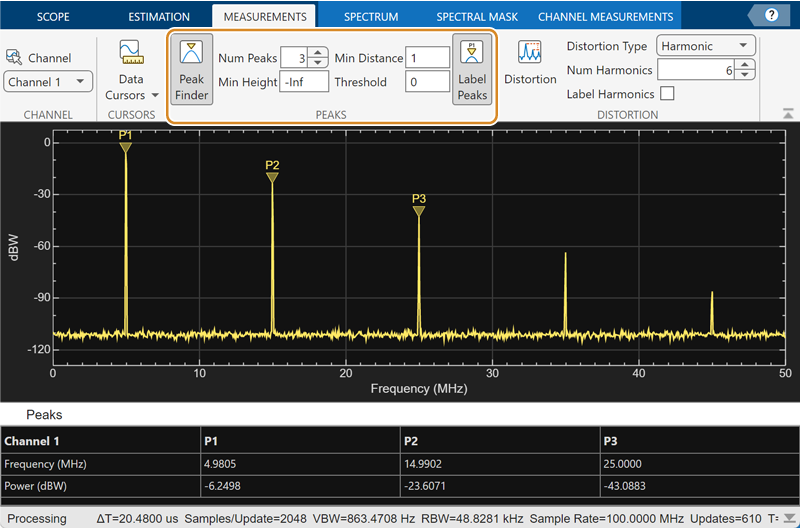Time Scope Toolstrip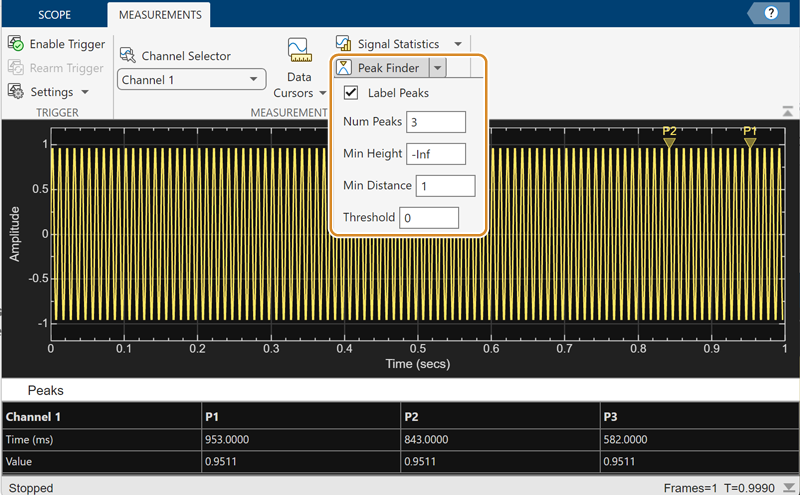Array Plot Toolstrip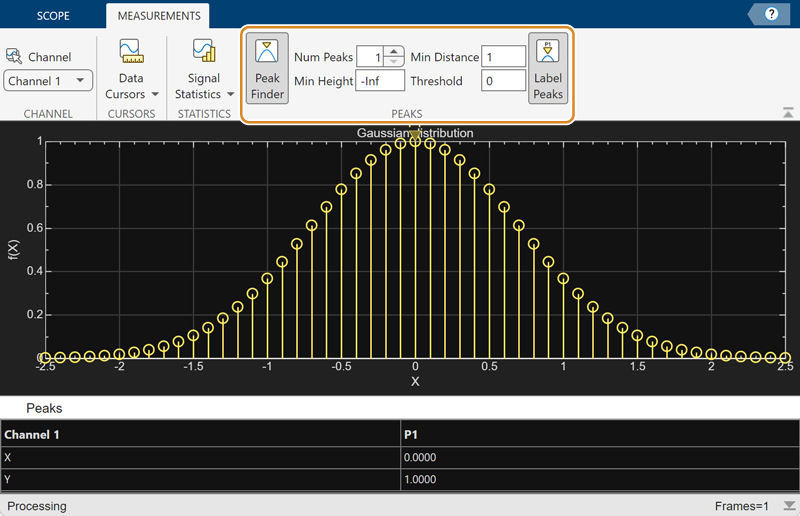Dynamic Filter Visualizer Toolstrip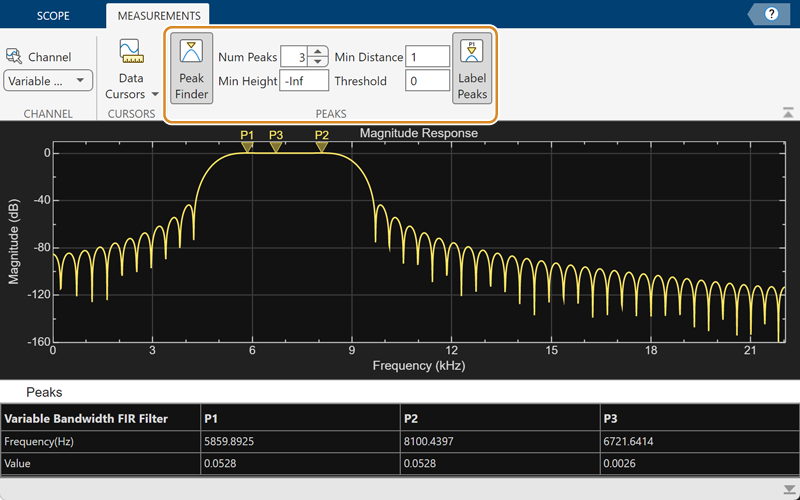## Creation

### Syntax

``pkfinder = PeakFinderConfiguration()``

### Description

example

````pkfinder = PeakFinderConfiguration()` creates a peak finder configuration object. ```

## Properties

expand all

All properties are tunable.

Level above which the scope detects peaks, specified as a real scalar.

#### Scope Window Use

On the Measurements tab, select Peak Finder. In the peak finder settings, specify a real scalar in the Min Height box.

Data Types: `double`

Maximum number of peaks to show, specified as a positive integer less than 100.

#### Scope Window Use

On the Measurements tab, select Peak Finder. In the peak finder settings, specify a positive integer less than 100 in the Num Peaks box.

Data Types: `double`

Minimum number of samples between adjacent peaks, specified as a positive integer.

#### Scope Window Use

On the Measurements tab, select Peak Finder. In the peak finder settings, specify a positive integer in the Min Distance box.

Data Types: `double`

Minimum difference in the height of the peak and its neighboring samples, specified as a nonnegative scalar.

#### Scope Window Use

On the Measurements tab, select Peak Finder. In the peak finder settings, specify a nonnegative scalar in the Threshold box.

Data Types: `double`

Label found peaks, specified as `true` or `false`. The scope displays the labels (P1, P2, …) above the arrows in the plot.

#### Scope Window Use

On the Measurements tab, select Peak Finder. In the peak finder settings, select Label Peaks.

Data Types: `logical`

Coordinates to display next to the calculated peak value, specified as `"x"`, `"y"`, or `"x + y"`.

Data Types: `char` | `string`

Enable peak finder measurements, specified as `true` or `false`. Set this property to `true` to enable the peak finder measurements.

#### Scope Window Use

On the Measurements tab, select Peak Finder.

Data Types: `logical`

## Examples

collapse all

Create a sine wave and view it in the Time Scope. Enable the peak finder programmatically.

Initialization

Create the input sine wave using the `sin` function. Create a `timescope` MATLAB® object to display the signal. Set the `TimeSpan` property to 1 second.

```f = 100; fs = 1000; swv = sin(2.*pi.*f.*(0:1/fs:1-1/fs)).'; scope = timescope(SampleRate=fs,... TimeSpanSource="property", ... TimeSpan=1);```

Peaks

Enable the peak finder and label the peaks. Set the scope to show three peaks and label them.

```scope.PeakFinder.Enabled = true; scope.PeakFinder.LabelPeaks = true; scope(swv) release(scope)```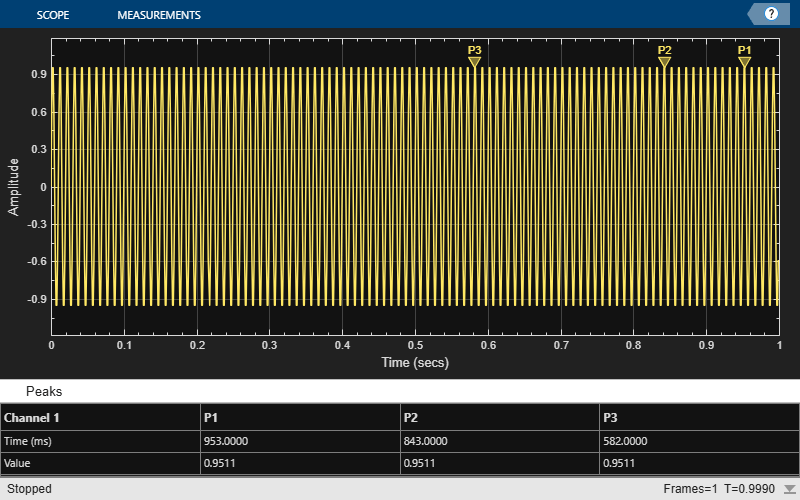Compute and display the power spectrum of a noisy sinusoidal input signal using the `spectrumAnalyzer` MATLAB® object. Measure the peaks, cursor placements, adjacent channel power ratio, and distortion values in the spectrum by enabling these properties:

• `PeakFinder`

• `CursorMeasurements`

• `ChannelMeasurements`

• `DistortionMeasurements`

Initialization

The input sine wave has two frequencies: 1000 Hz and 5000 Hz. Create two `dsp.SineWave` System objects to generate these two frequencies. Create a `spectrumAnalyzer` object to compute and display the power spectrum.

```Fs = 44100; Sineobject1 = dsp.SineWave(SamplesPerFrame=1024,PhaseOffset=10,... SampleRate=Fs,Frequency=1000); Sineobject2 = dsp.SineWave(SamplesPerFrame=1024,... SampleRate=Fs,Frequency=5000); SA = spectrumAnalyzer(SampleRate=Fs,SpectrumType="power",... PlotAsTwoSidedSpectrum=false,ChannelNames={'Power spectrum of the input'},... YLimits=[-120 40],ShowLegend=true);```

Enable Measurements Data

To obtain the measurements, set the `Enabled` property to `true`.

```SA.CursorMeasurements.Enabled = true; SA.ChannelMeasurements.Enabled = true; SA.PeakFinder.Enabled = true; SA.DistortionMeasurements.Enabled = true;```

Use `getMeasurementsData`

Stream in the noisy sine wave input signal and estimate the power spectrum of the signal using the `spectrumAnalyzer` object. Measure the characteristics of the spectrum. Use the `getMeasurementsData` function to obtain these measurements programmatically. The `isNewDataReady` function returns `true` when there is new spectrum data. Store the measured data in the variable `data`.

```data = []; for Iter = 1:1000 Sinewave1 = Sineobject1(); Sinewave2 = Sineobject2(); Input = Sinewave1 + Sinewave2; NoisyInput = Input + 0.001*randn(1024,1); SA(NoisyInput); if SA.isNewDataReady data = [data;getMeasurementsData(SA)]; end end```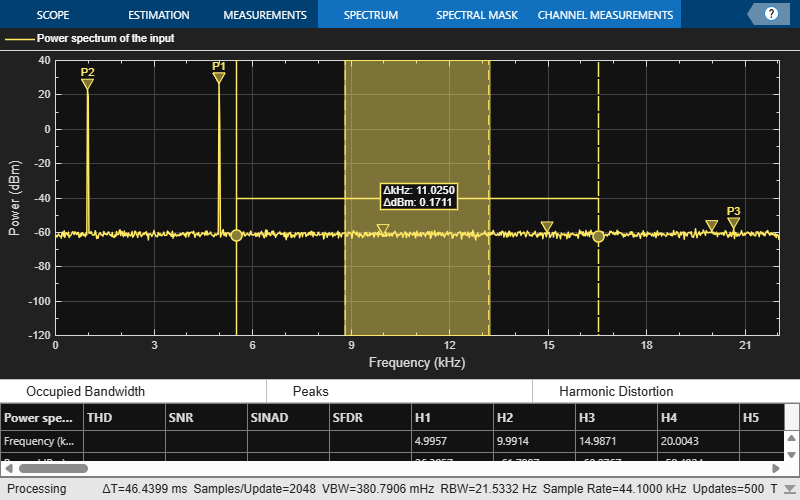The panes at the bottom of the scope window display the measurements that you have enabled. The values in these panes match the values in the last time step of the `data` variable. You can access the individual fields of `data` to obtain the various measurements programmatically.

Compare Peak Values

Use the `PeakFinder` property to obtain peak values. Verify that the peak values in the last time step of `data` match the values in the spectrum analyzer plot.

`peakvalues = data.PeakFinder(end).Value `
```peakvalues = 3×1 26.3957 22.7830 -57.9977 ```
`frequencieskHz = data.PeakFinder(end).Frequency/1000`
```frequencieskHz = 3×1 4.9957 0.9905 20.6719 ```

## Version History

Introduced in R2022a

expand all